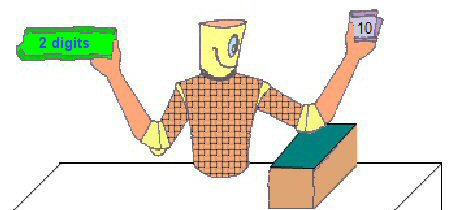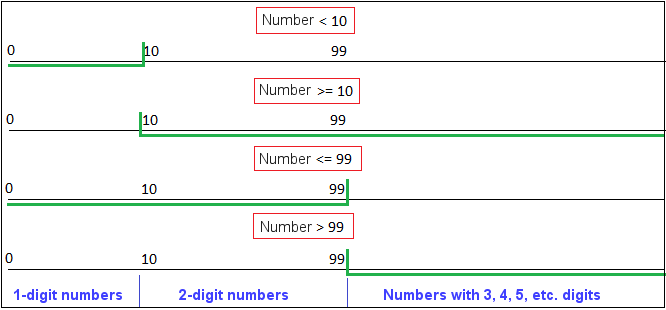# JavaScript : Boolean Expressions I

Objectives

1. Construct Boolean expressions using AND and OR.Write your code so that the robot correctly shows how many digits there are in the number it picks.

The robot can pick a random number between 5 and 120. It must then check whether or not it is a 2-digit number, and show the result.

There are 4 tests it can use to do this:

1. lessThan10() checks whether the number is less than 10;
2. greaterOrEqual10() checks if it is greater than or equal to 10;
3. lessOrEqual99() checks if it is less than or equal to 99;
4. greaterThan99() checks if it is greater than 99.

Working with « Ranges »

The goal is to separate the 2-digit numbers from the numbers that are not 2 digits using the least amount of code possible. You are not to use « else » and since there are just 2 possibilities (for the number to be a 2-digit number or not), it should be possible to achieve this with two successive « if » statements.

When working with values, it is often helpful to view ranges graphically. For example, in the graphic below, the ranges in green correspond to the available tests. The areas in blue show how many digits the numbers have.This makes it fairly clear that the areas in which the numbers are not 2-digit numbers are the union of the entire area of lessThan10() and the entire area of greaterThan99().

It is also clear that the area in which the numbers do have 2 digits are the intersection of the entire area of greaterOrEqual10() and the entire area of lessOrEqual99().

Now you just need to translate this into logical expressions, then write your code using the correct syntax, and you'll be all set…

Refer to the chapter on theory just before this one if this is not yet clear to you.

Good luck!

Back